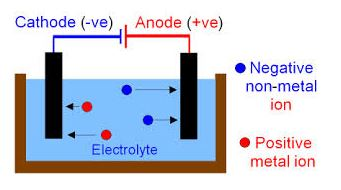Physic Tutorials

# Notes on conduction through liquid and gases

Notes on conduction through liquid and gases

CONDUCTION THROUGH LIQUIDS

Electrolysis: Is the chemical change in a liquid due to the flow of electric current.

Electrolytes Are liquid that are good conductor of electricity.

Non-electrolytes are liquid that are poor conductor of electricity.Application of Electrolysis

1. Electroplating: Is the process of coating metals with another metal in order to protect the metal from corrosion.
2. Purification of Metal: Electrolysis is important in purifying impure copper.

Faraday’s First Law: States that the mass of a substance, M, of a substance liberated during electrolysis is strictly proportional to the quantity of electricity that has passed through the electrolyte.

Therefore:

M      =        Mass of a substance

Q       =        Quantity of electricity

M      =        zIt

Q = It

where z constant of proportionality which is the electrochemical equivalence

Faraday’s Second Law: States that he masses of the different substance deposited or liberated by the same quantity of electricity are strictly proportional to the equivalent of the substance.

Example 1

At what time must a current of 20A pass through a solution fo zinc sulphate to deposit 3g of zinc? If the electrochemical equivalent z = 0.0003387gc^-1.

I        =        20A

m = 3g

Z       =        0.0003387

M      =        zit

t        =        M/ ZI

t        =     3 / 0.0003387*20

t        =        443s

Gases

Conditions under which gases conduct electricity

1. Low pressure
2. High potential difference

Thermionic Emission

Thermionic emission: This is the emission of electrons from the surface of a hot metal. Whenever a metal is heated to a greater temperature, electrons are emitted from the surface of the metal in a process known as thermionic emission.

Application of thermionic emission

The application of thermionic emission is in the cathode –ray oscilloscope used for studying all types of waveforms, it is also important in measuring frequencies and amplitudes of voltages of electronic devices.

Recommended: Solved questions in physics

### Bolarinwa Olajire

A lecturer, Educationist, PhD student at FUNAAB, and a Blogger.

### One Comment

1.Nkeonye frank says:

I love this study page